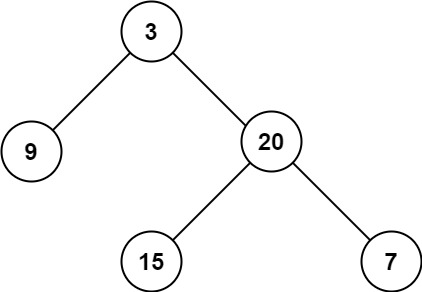# 104.maximum-depth-of-binary-tree

## Statement

• Difficulty: Easy
• Tag: `树` `深度优先搜索` `广度优先搜索` `二叉树`

``````    3
/
9  20
/
15   7``````

• Link: Maximum Depth of Binary Tree
• Difficulty: Easy
• Tag: `Tree` `Depth-First Search` `Breadth-First Search` `Binary Tree`

Given the `root` of a binary tree, return its maximum depth.

A binary tree's maximum depth is the number of nodes along the longest path from the root node down to the farthest leaf node.

Example 1:``````Input: root = [3,9,20,null,null,15,7]
Output: 3
``````

Example 2:

``````Input: root = [1,null,2]
Output: 2
``````

Constraints:

• The number of nodes in the tree is in the range `[0, 104]`.
• `-100 <= Node.val <= 100`

## Solution

``````# Definition for a binary tree node.
# class TreeNode:
#     def __init__(self, val=0, left=None, right=None):
#         self.val = val
#         self.left = left
#         self.right = right
from typing import Optional

class Solution:
res = 0

def dfs(self, rt: TreeNode, d: int) -> None:
if not rt:
return

cur_d = d + 1
self.res = max(self.res, cur_d)
self.dfs(rt.left, cur_d)
self.dfs(rt.right, cur_d)

def maxDepth(self, root: Optional[TreeNode]) -> int:
self.dfs(root, 0)
return self.res
``````